# write a formal proof and state witch proof style you use 1 1 + +...+ 3.4...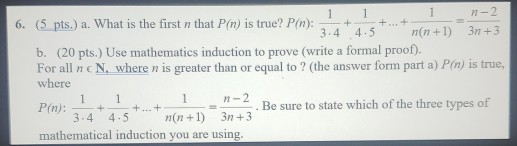write a formal proof and state witch proof style you use

1 1 + +...+ 3.4 n-2 6. (5 pts.) a. What is the first n that P(n) is true? P(n): 4.5 n(n+1) 3n+3 b. (20 pts. Use mathematics induction to prove (write a formal proof). For all ne N, where n is greater than or equal to? (the answer form part a) P(n) is true, where 1 1-2 P(n): Be sure to state which of the three types of 3.4 4.5 n(n+1) 3n+3 mathematical induction you are using. 1 1 + + ... +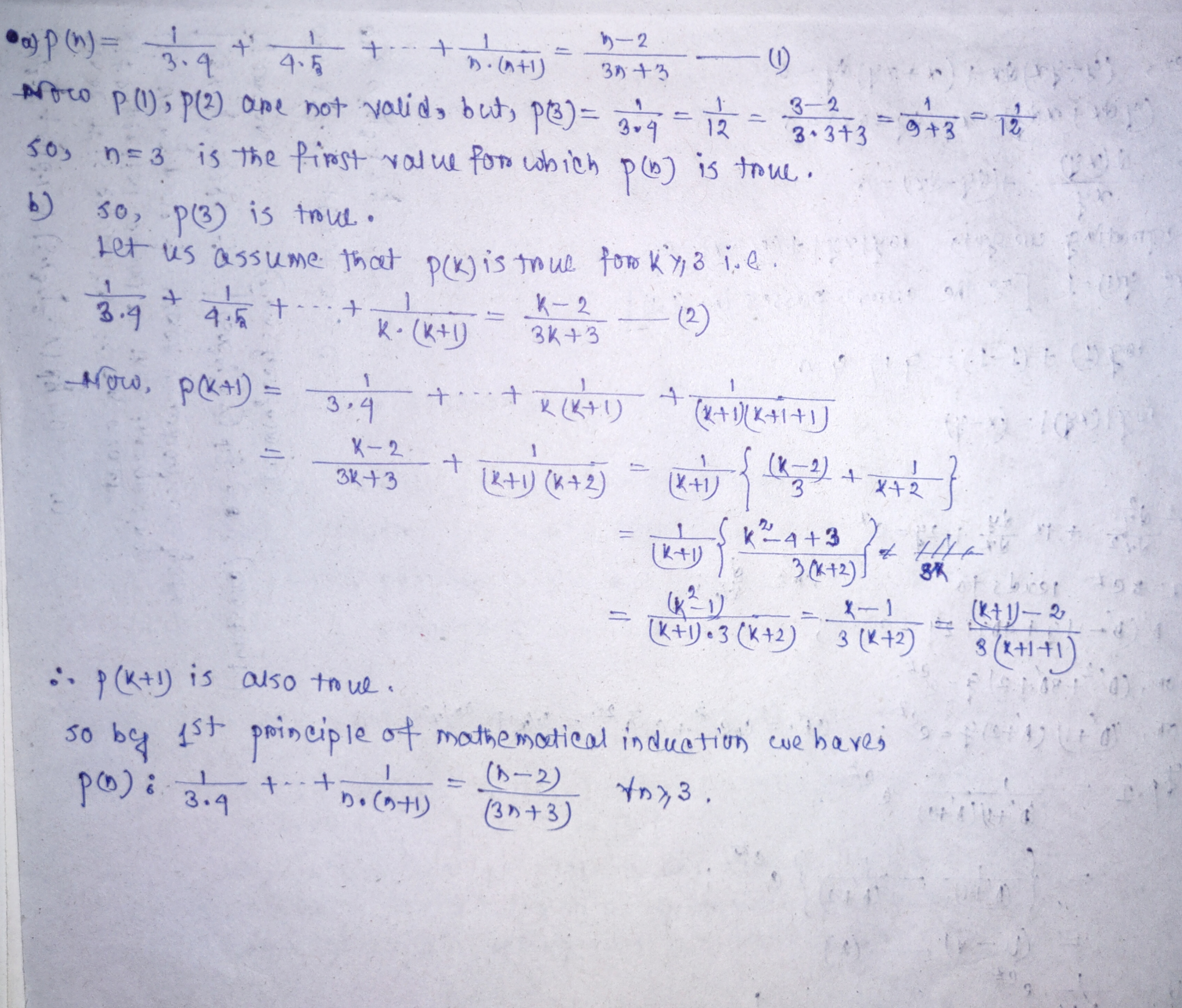#### Earn Coin

Coins can be redeemed for fabulous gifts.

Similar Homework Help Questions
• ### Write a formal proof to prove the following conjecture to be true or false. If the...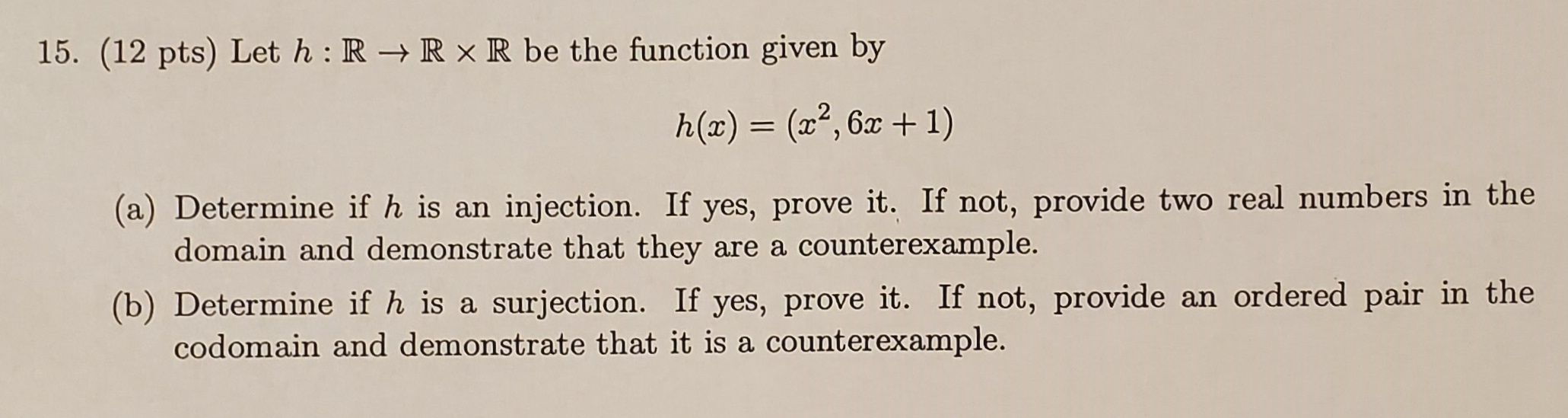Write a formal proof to prove the following conjecture to be true or false. If the statement is true, write a formal proof of it. If the statement is false, provide a counterexample and a slightly modified statement that is true and write a formal proof of your new statement. Conjecture: 15. (12 pts) Let h: R + RxR be the function given by h(x) = (x²,6x + 1) (a) Determine if h is an injection. If yes, prove it....

• ### Problem #5 [30 Points) Prove each of the following statements by Mathematical Induction. Make sure you...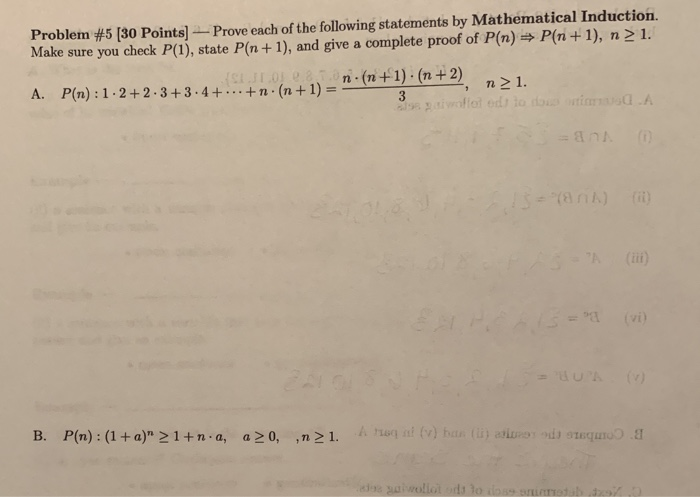Problem #5 [30 Points) Prove each of the following statements by Mathematical Induction. Make sure you check P(1), state P(n+1), and give a complete proof of P(n) = P(n+1), n > 1. A. n.(n+1)(n+2) n 1. P(n): 1. 2+2-3+3.4+...+n·(n + 1) = - to od to be used B. P(n): (1 + a)" > 1+na, a>0,,n>1. ) han de stem

• ### Question 3 You should be able to answer this question after studying Unit 9. (a) Use...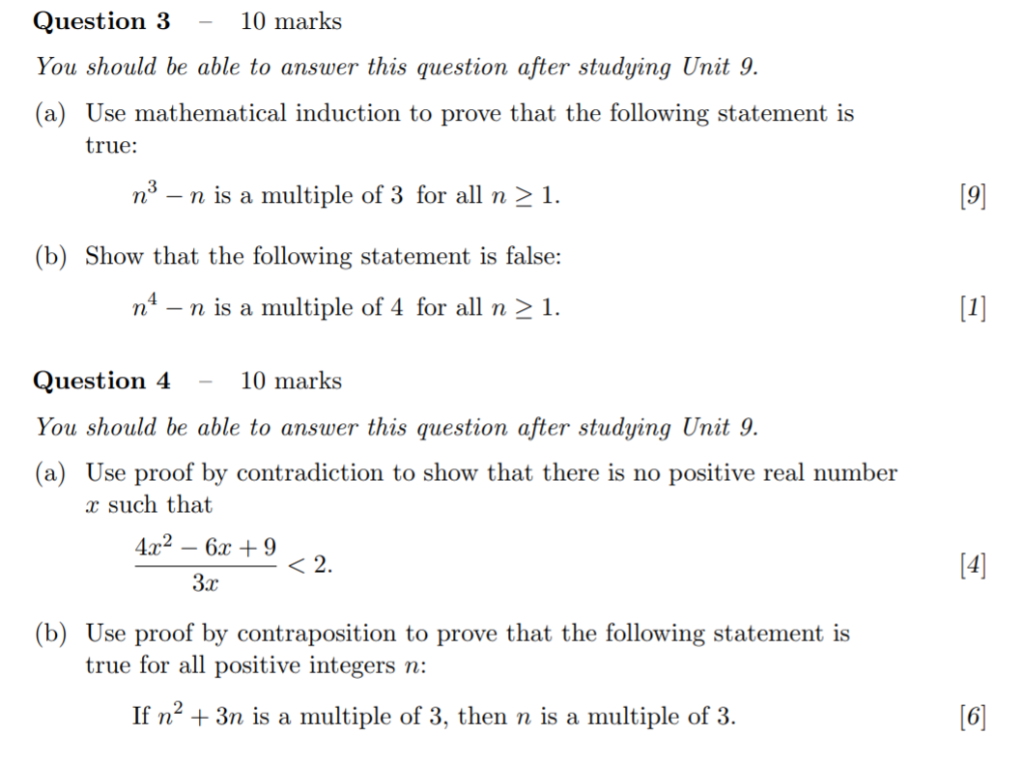Question 3 You should be able to answer this question after studying Unit 9. (a) Use mathematical induction to prove that the following statement is 10 marks true: [9 n - n is a multiple of 3 for all n 2 1. (b) Show that the following statement is false: n-n is a multiple of 4 for all n 2 1. Question 4-10 ma You should be able to answer this question after studying Unit 9. (a) Use proof by...

• ### (a) Suppose you wish to use the Principle of Mathematical Induction to prove that n(n+1) 1+...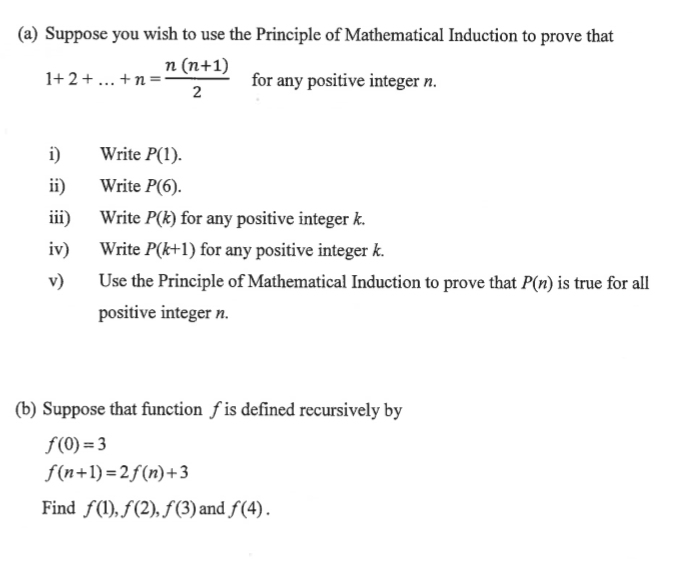(a) Suppose you wish to use the Principle of Mathematical Induction to prove that n(n+1) 1+ 2+ ... +n= - for any positive integer n. i) Write P(1). Write P(6. Write P(k) for any positive integer k. Write P(k+1) for any positive integer k. Use the Principle of Mathematical Induction to prove that P(n) is true for all positive integer n. (b) Suppose that function f is defined recursively by f(0) = 3 f(n+1)=2f (n)+3 Find f(1), f (2), f...

• ### Problem 1 148pts] (1) I 10pts! Let P(n) be the statement that l + 2 +...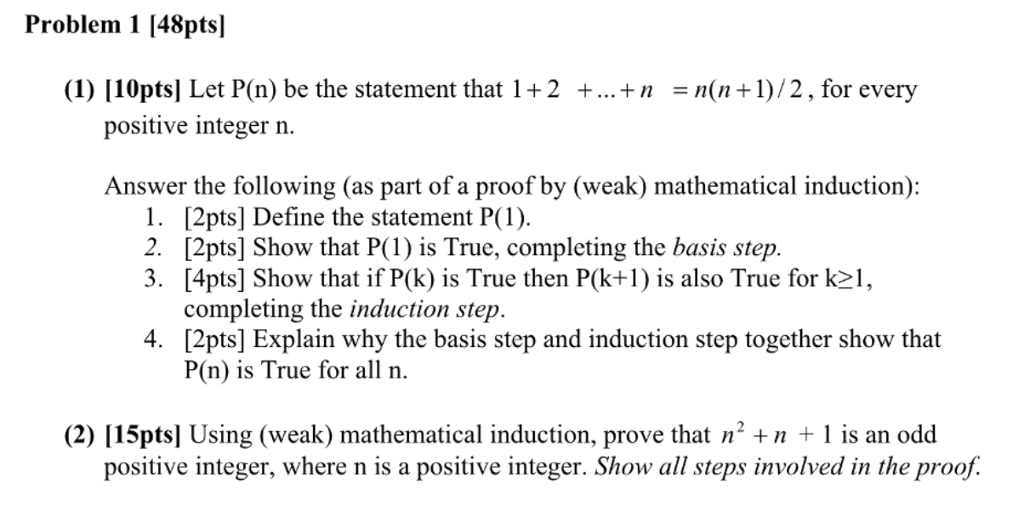Problem 1 148pts] (1) I 10pts! Let P(n) be the statement that l + 2 + + n n(n + 1) / 2 , for every positive integer n. Answer the following (as part of a proof by (weak) mathematical induction): 1. [2pts] Define the statement P(1) 2. [2pts] Show that P(1 is True, completing the basis step. 3. [4pts] Show that if P(k) is True then P(k+1 is also True for k1, completing the induction step. [2pts] Explain why...

• ### 11: I can identify the predicate being used in a proof by mathematical induction and use...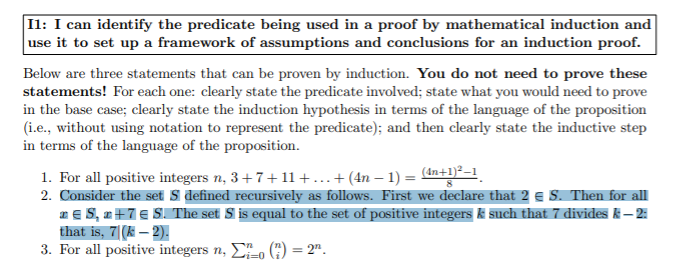11: I can identify the predicate being used in a proof by mathematical induction and use it to set up a framework of assumptions and conclusions for an induction proof. Below are three statements that can be proven by induction. You do not need to prove these statements! For each one clearly state the predicate involved; state what you would need to prove in the base case; clearly state the induction hypothesis in terms of the language of the proposition...

• ### 2: Use mathematical induction to prove that for any odd integer n >= 1, 4 divides...

2: Use mathematical induction to prove that for any odd integer n >= 1, 4 divides 3n + 1 ====== Please type / write clearly. Thank you, and I will thumbs up!

• ### Main topic and problems for the final project The main purpose of the project is to introduce you how to use a in a...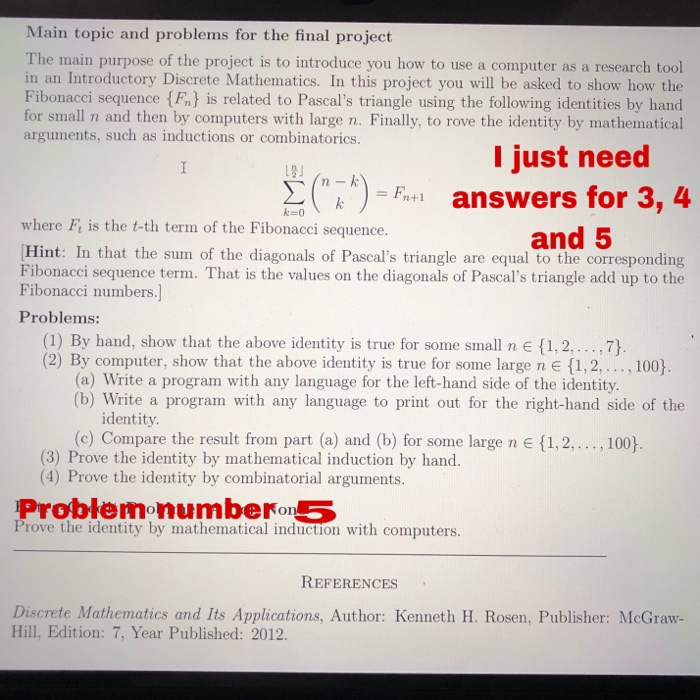Main topic and problems for the final project The main purpose of the project is to introduce you how to use a in an computer as a research tool Introductory Discrete Mathematics. In this project you will be asked to show how the Fibonacci sequence {Fn} is related to Pascal's triangle using the following identities by hand for small and then by computers with large n. Finally, to rove the identity by mathematical arguments, such as inductions or combinatorics. I...

• ### 1. Use mathematical induction to prove ZM-1), in Ik + 6 for integers n and k...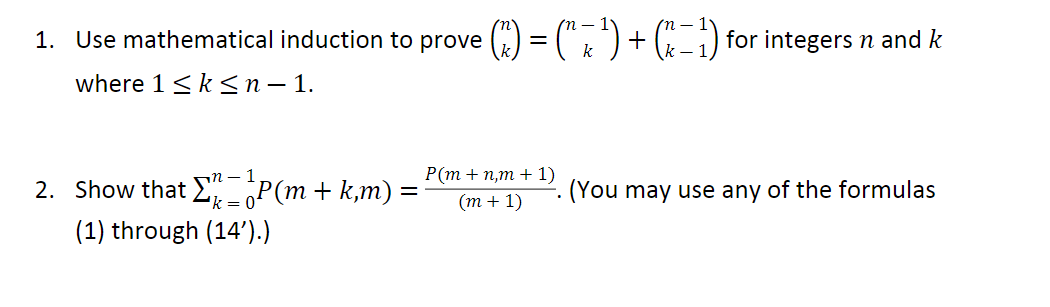1. Use mathematical induction to prove ZM-1), in Ik + 6 for integers n and k where 1 <k<n - 1. = 2. Show that I" - P(m + k,m) = P(m+n,m+1) (m + 1) F. (You may use any of the formulas (1) through (14”).)

• ### Use mathematical induction to prove that the statement is true for every positive integer n. 1'3+...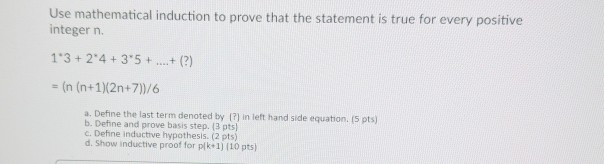Use mathematical induction to prove that the statement is true for every positive integer n. 1'3+ 24 +3'5 +...+() = (n (n+1)(2n+7))/6 a. Define the last term denoted by t) in left hand side equation. (5 pts) b. Define and prove basis step. 3 pts c. Define inductive hypothesis (2 pts) d. Show inductive proof for pik 1) (10 pts)